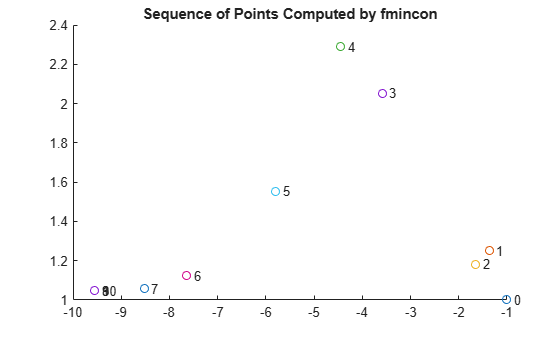Documentation

## Output Function for Problem-Based Optimization

This example shows how to use an output function to plot and store the history of the iterations for a nonlinear problem. This history includes the evaluated points, the search directions that the solver uses to generate points, and the objective function values at the evaluated points.

For the solver-based approach to this example, see Output Functions.

Plot functions have the same syntax as output functions, so this example also applies to plot functions, too.

For both the solver-based approach and for the problem-based approach, write the output function as if you are using the solver-based approach. In the solver-based approach, you use a single vector variable, usually denoted `x`, instead of a collection of optimization variables of various sizes. So to write an output function for the problem-based approach, you must understand the correspondence between your optimization variables and the single solver-based `x`. To map between optimization variables and `x`, use `varindex`. In this example, to avoid confusion with an optimization variable named `x`, use "`in"` as the vector variable name.

### Problem Description

The problem is to minimize the following function of variables `x` and `y`:

`$f=\mathrm{exp}\left(x\right)\left(4{x}^{2}+2{y}^{2}+4xy+2y+1\right).$`

In addition, the problem has two nonlinear constraints:

`$\begin{array}{l}x+y-xy\ge 1.5\\ xy\ge 10.\end{array}$`

### Problem-Based Setup

To set up the problem in the problem-based approach, define optimization variables and an optimization problem object.

```x = optimvar('x'); y = optimvar('y'); prob = optimproblem;```

The objective function is in the `objfun.m` file.

`type objfun`
```function f = objfun(x,y) %OBJFUN Objective function. % Documentation example. % Copyright 2018 The MathWorks, Inc. f = exp(x) * (4*x^2 + 2*y^2 + 4*x*y + 2*y + 1); ```

This expression is not a rational function of its variables. Therefore, to include it in the objective, first convert the function to an optimization expression by using `fcn2optimexpr`.

`prob.Objective = fcn2optimexpr(@objfun,x,y);`

To include the nonlinear constraints, create optimization constraint expressions.

```cons1 = x + y - x*y >= 1.5; cons2 = x*y >= -10; prob.Constraints.cons1 = cons1; prob.Constraints.cons2 = cons2;```

Because this is a nonlinear problem, you must include an initial point structure `x0`. Use `x0.x = –1` and `x0.y = 1`.

```x0.x = -1; x0.y = 1;```

### Output Function

The `outfun` output function records a history of the points generated by `fmincon` during its iterations. The output function also plots the points and keeps a separate history of the search directions for the `sqp` algorithm. The search direction is a vector from the previous point to the next point that `fmincon` tries. During its final step, the output function saves the history in workspace variables, and saves a history of the objective function values at each iterative step.

For the required syntax of optimization output functions, see Output Function Syntax.

An output function takes a single vector variable as an input. But the current problem has two variables. To find the mapping between the optimization variables and the input variable, use `varindex`.

```idx = varindex(prob); idx.x```
```ans = 1 ```
`idx.y`
```ans = 2 ```

The mapping shows that `x` is variable 1 and `y` is variable 2. So, if the input variable is named `in`, then `x = in(1)` and `y = in(2)`.

`type outfun`
```function stop = outfun(in,optimValues,state,idx) persistent history searchdir fhistory stop = false; switch state case 'init' hold on history = []; fhistory = []; searchdir = []; case 'iter' % Concatenate current point and objective function % value with history. in must be a row vector. fhistory = [fhistory; optimValues.fval]; history = [history; in(:)']; % Ensure in is a row vector % Concatenate current search direction with % searchdir. searchdir = [searchdir;... optimValues.searchdirection(:)']; plot(in(idx.x),in(idx.y),'o'); % Label points with iteration number and add title. % Add .15 to idx.x to separate label from plotted 'o' text(in(idx.x)+.15,in(idx.y),... num2str(optimValues.iteration)); title('Sequence of Points Computed by fmincon'); case 'done' hold off assignin('base','optimhistory',history); assignin('base','searchdirhistory',searchdir); assignin('base','functionhistory',fhistory); otherwise end end ```

Include the output function in the optimization by setting the `OutputFcn` option. Also, set the `Algorithm` option to use the `'sqp'` algorithm instead of the default `'interior-point'` algorithm. Pass `idx` to the output function as an extra parameter in the last input. See Passing Extra Parameters.

```outputfn = @(in,optimValues,state)outfun(in,optimValues,state,idx); opts = optimoptions('fmincon','Algorithm','sqp','OutputFcn',outputfn);```

### Run Optimization Using Output Function

Run the optimization, including the output function, by using the '`Options'` name-value pair argument.

`[sol,fval,eflag,output] = solve(prob,x0,'Options',opts)`
```Solving problem using fmincon. ``````Local minimum found that satisfies the constraints. Optimization completed because the objective function is non-decreasing in feasible directions, to within the value of the optimality tolerance, and constraints are satisfied to within the value of the constraint tolerance. ```
```sol = struct with fields: x: -9.5474 y: 1.0474 ```
```fval = 0.0236 ```
```eflag = OptimalSolution ```
```output = struct with fields: iterations: 10 funcCount: 34 algorithm: 'sqp' message: '...' constrviolation: 0 stepsize: 1.4787e-07 lssteplength: 1 firstorderopt: 1.0231e-09 solver: 'fmincon' ```

Examine the iteration history. Each row of the `optimhistory` matrix represents one point. The last few points are very close, which explains why the plotted sequence shows overprinted numbers for points 8, 9, and 10.

`disp('Locations');disp(optimhistory)`
```Locations -1.0000 1.0000 -1.3679 1.2500 -1.6509 1.1813 -3.5870 2.0537 -4.4574 2.2895 -5.8015 1.5531 -7.6498 1.1225 -8.5223 1.0572 -9.5463 1.0464 -9.5474 1.0474 -9.5474 1.0474 ```

Examine the `searchdirhistory` and `functionhistory` arrays.

`disp('Search Directions');disp(searchdirhistory)`
```Search Directions 0 0 -0.3679 0.2500 -0.2831 -0.0687 -1.9360 0.8725 -0.8704 0.2358 -1.3441 -0.7364 -2.0877 -0.6493 -0.8725 -0.0653 -1.0241 -0.0108 -0.0011 0.0010 0.0000 -0.0000 ```
`disp('Function Values');disp(functionhistory)`
```Function Values 1.8394 1.8513 1.7757 0.9839 0.6343 0.3250 0.0978 0.0517 0.0236 0.0236 0.0236 ```